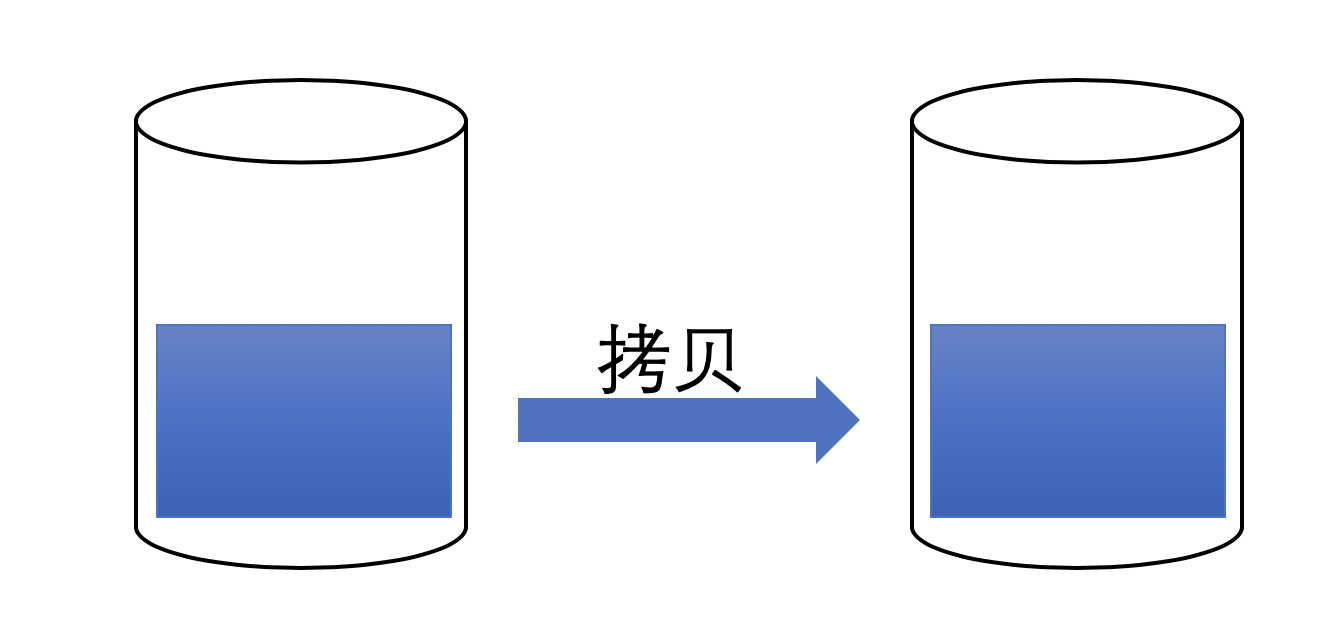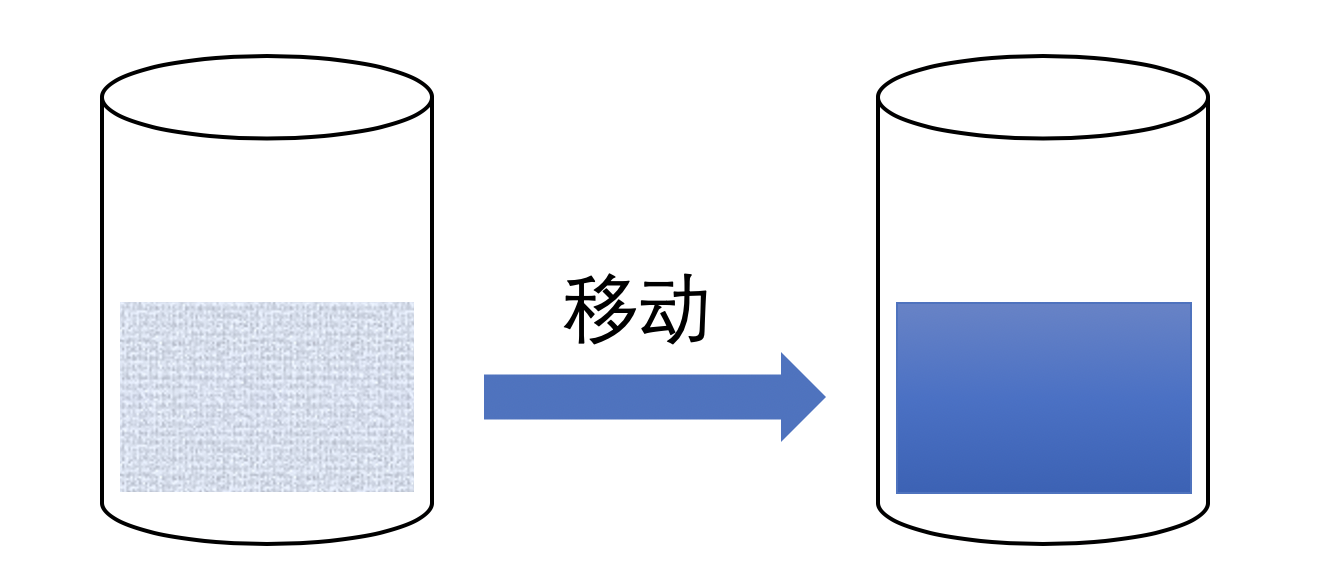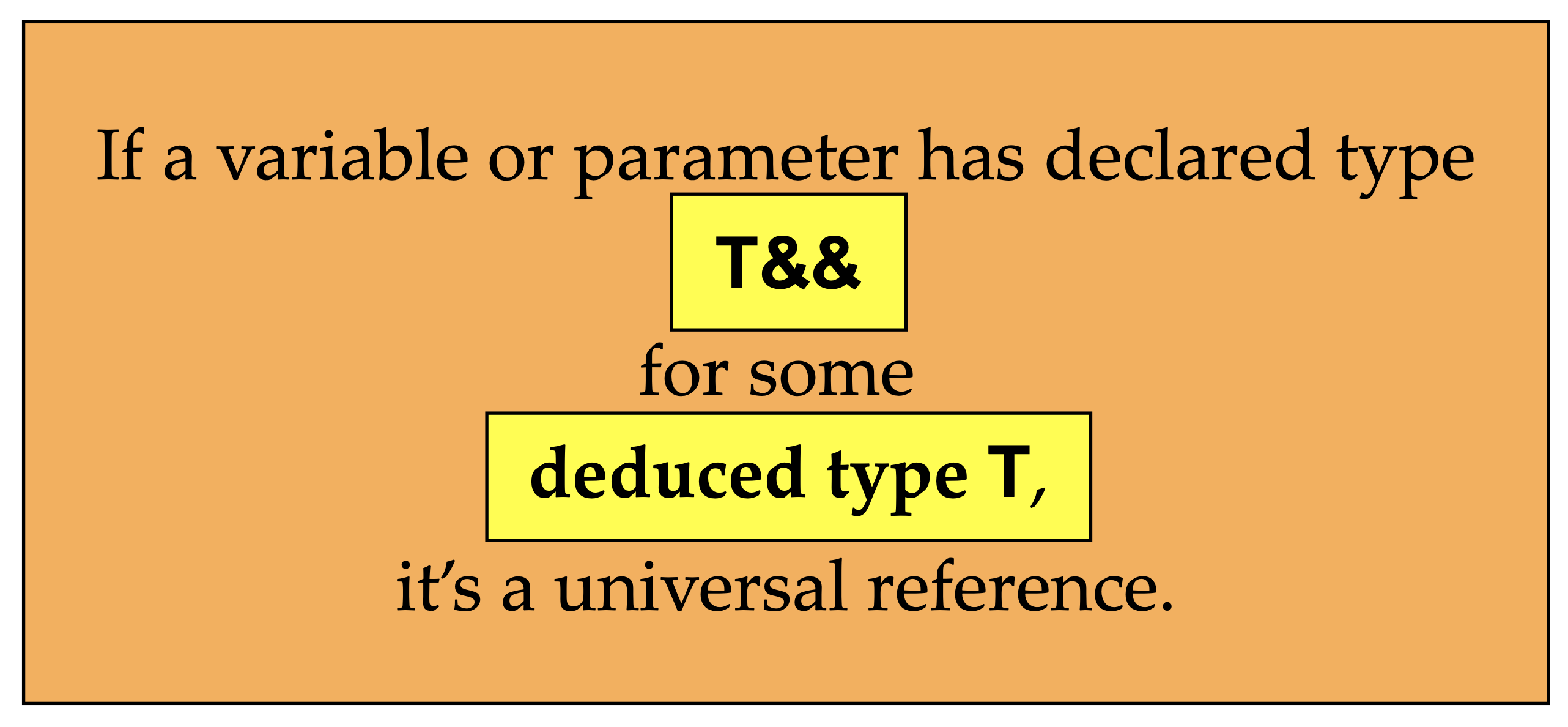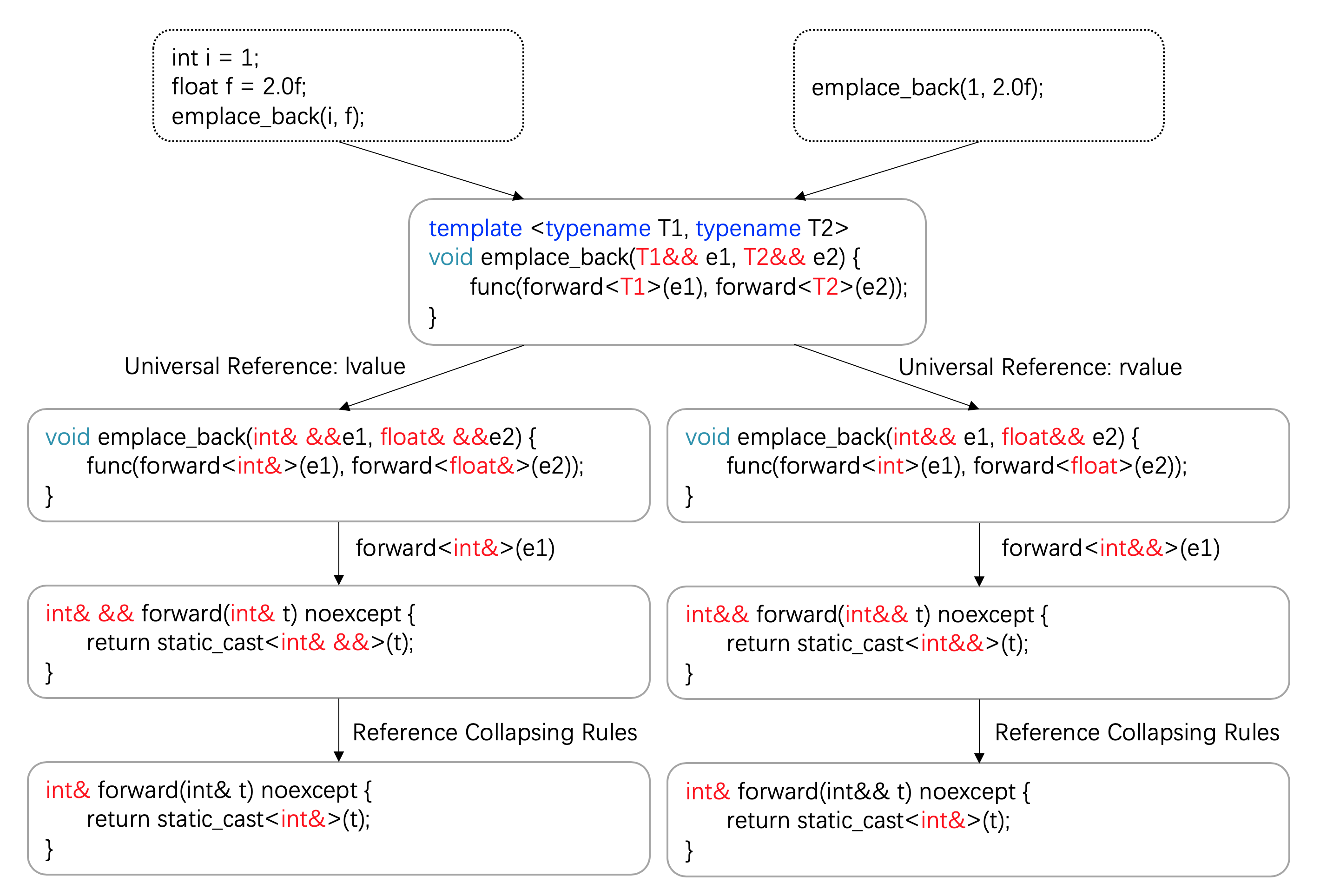## C++中的值类别

Posted on Jul 12, 2020# 表达式与值类别

C++的程序由一系列的表达式（expressions）构成。表达式是运算符和操作数的序列，表达式指定一项计算。

• 类型（type）。类型是我们很熟悉的概念，intdoublestd::string这些都是类型。类型确定了表达式可以进行哪些操作。
• 除了类型之外，还有一个称之为值类别（value category）的属性，却可能是我们平时不太注意的。

type和category在中文中似乎都可以翻译成“类型”。但在本文中，为了区分它们，统一将type翻译成“类型”，category翻译成“类别”。

# 为什么要懂这些东西？

 error: lvalue required as left operand of assignment


# 从左值和右值说起

lvalue之所以叫lvalue，是因为它常常出现在等号的左边（left-hand side of an assignment）。同样，rvalue是因为它常常出现在等号的右边（right-hand side of an assignment）。

C语言遵循了相似的分类法，但是否需要等号赋值已经不再重要。在C语言中，标识一个对象的表达式称之为左值，不过lvalue已经是“locator value”的简写，因为lvalue对应了一块内存地址。

# C++11中的值类别

C++中对于值类别的定义也经历一些变化。从C++11标准开始，值类别早以不止是lvalue和rvalue两种这么简单。

• A glvalue（generalized lvalue） is an expression whose evaluation determines the identity of an object, bit-field, or function.
• A prvalue（pure rvalue） is an expression whose evaluation initializes an object or a bit-field, or computes the value of an operand of an operator, as specified by the context in which it appears, or an expression that has type cv void.
• An xvalue（eXpiring value） is a glvalue that denotes an object or bit-field whose resources can be reused （usually because it is near the end of its lifetime）.
• An lvalue is a glvalue that is not an xvalue.
• An rvalue is a prvalue or an xvalue.

• 是否拥有身份（identity）：可以确定表达式是否与另一表达式指代同一实体，例如比较它们所标识的对象或函数的（直接或间接获得的）地址；
• 是否可被移动（具体见下文）：移动构造函数、移动赋值运算符或实现了移动语义的其他函数重载能够绑定到这个表达式。

• lvalue是指：拥有身份且不可被移动的表达式。
• xvalue是指：拥有身份且可被移动的表达式。
• prvalue是指：不拥有身份且可被移动的表达式。
• glvalue是指：拥有身份的表达式，lvalue和xvalue都是glvalue。
• rvalue是指：可被移动的表达式。prvalue和xvalue都是rvalue。

拥有身份（glvalue） 不拥有身份## glvalue

glvalue是拥有身份的表达式，它对应了一块内存地址。glvalue有lvalue和xvalue两种形式，具体的示例见下文。

glvalue具有以下一些特性：

• glvalue可以自动转换成prvalue。例如：int a = b，等号右边的lvalue会自动转换成rvalue。
• glvalue可以是多态的（polymorphic），它所对应了动态类型和静态类型可以不一样，例如：一个指向子类的父类指针。
• glvalue可以是不完整类型，只要表达式允许。例如：由前置声明但未定义的类类型。

## rvalue

rvalue是指可以移动的表达式。prvalue和xvalue都是rvalue，具体的示例见下文。

rvalue具有以下特征：

• 无法对rvalue进行取地址操作。例如：&42&i++，这些表达式没有意义，也编译不过。
• rvalue不能放在赋值或者组合赋值符号的左边，例如：3 = 53 += 5，这些表达式没有意义，也编译不过。
• rvalue可以用来初始化const左值引用（见下文）。例如：const int& a = 1
• rvalue可以用来初始化右值引用（见下文）。
• rvalue可以影响函数重载：当被用作函数实参且该函数有两种重载可用，其中之一接受右值引用的形参而另一个接受 const 的左值引用的形参时，右值将被绑定到右值引用的重载之上。

## lvalue

"hello world" // lvalue
int a{}; // lvalue
++a; // lvalue

int& get() {return a;}
get(); // lvalue

int b{}; // lvalue
b; // lvalue

int foo();
int &&a { foo() }; // lvalue

struct foo {int a;};
foo f; // lvalue
f.a; // lvalue

int &&c{ 55 }; // lvalue
int &d{ a }; // lvalue


lvalue具有以下特征：

• 所有glvalue具有的特征
• 可以通过取址运算符获取其地址
• 可修改的左值可用作内建赋值和内建符合赋值运算符的左操作数
• 可以用来初始化左值引用（见下文）

## prvalue

prvalue是纯右值，数字字面值或者函数返回的是非引用的值都是prvalue。

42 // prvalue
true // prvalue

int foo();
foo();// prvalue

int a{}, b{}; // both lvalues
a + b; // prvalue
&a; // prvalue

a++ // prvalue
b-- // prvalue
a && b  // prvalue
a < b // prvalue

double {}; // prvalue
std::vector<X> {}; // prvalue


prvalue具有以下特征：

• 所有rvalue具有的特征
• prvalue不会是多态的
• prvalue不会是不完全类型
• prvalue不会是抽象类型或数组

## xvalue

xvalue也指向了一个对象，不过这个对象已经接近了生命周期的末尾。这通常和移动语义（见下文）有关。

xvalue与右值引用有很强的关联性，因此看了下文对于右值引用的说明再回过头来看xvalue会更好理解。

bool b {true}; // lvalue
std::move(b); // xvalue
static_cast<bool&&>(b); // xvalue

int&& foo();
foo(); // xvalue

struct foo {int a;};
std::move(f).a; // xvalue
foo{}.a; // xvalue

int a{};
std::move(a); // xvalue
std::move(a); // xvalue

using arr = int;
arr{}; // xvalue


xvalue具有所有rvalue和glvalue所有的特征。

# 左值引用与右值引用

int& a = 10;


error: non-const lvalue reference to type 'int' cannot bind to a temporary of type 'int'


const int& a = 10;


C++11新增了右值引用，左值引用的写法是&，右值引用的写法是&&

std::string s1 = "Hello ";
std::string s2 = "world";
std::string&& s_rref = s1 + s2;    // the result of s1 + s2 is an rvalue
s_rref += ", my friend";           // I can change the temporary string!
std::cout << s_rref << '\n';       // prints "Hello world, my friend"


void func(X& x) {
cout << "lvalue reference version" << endl;
}

void func(X&& x) {
cout << "rvalue reference version" << endl;
}


X returnX() {
return X();
}

int main(int argc, char** argv) {
X x;
func(x);
func(returnX());
}


lvalue reference version
rvalue reference version


• 左值引用：即可以绑定到左值（非const），也可以绑定到右值（const）
• 右值引用：只能绑定到右值

• 移动语义（Move Semantics）
• 完美转发（Perfect Forward）

# 移动语义

class X
{
public:
X(const X& other) // copy constructor
{
m_data = new int[other.m_size];
std::copy(other.m_data, other.m_data + other.m_size, m_data);
m_size = other.m_size;
}

X& operator=(X other) // copy assignment
{
if(this == &other) return *this;
delete[] m_data;
m_data = new int[other.m_size];
std::copy(other.m_data, other.m_data + other.m_size, m_data);
m_size = other.m_size;
return *this;
}

X& operator=(const X& other) // copy assignment
{
if(this == &other) return *this;
delete[] m_data;
m_data = new int[other.m_size];
std::copy(other.m_data, other.m_data + other.m_size, m_data);
m_size = other.m_size;
return *this;
}

private:
int*   m_data;
size_t m_size;
};X(X&&);
X& operator=(X&&);


X(X&& other)     // <-- rvalue reference in input
{
m_data = other.m_data;   // ①
m_size = other.m_size;
other.m_data = nullptr;  // ②
other.m_size = 0;
}

X& operator=(X&& other)     // <-- rvalue reference in input
{
if (this == &other) return *this;

delete[] m_data;         // ③

m_data = other.m_data;   // ④
m_size = other.m_size;

other.m_data = nullptr;  // ⑤
other.m_size = 0;

return *this;
}


1. 获取other对象所包含的值
2. 处理other的内部结构，防止再次使用
3. 释放自身包含的指针
4. 获取other对象所包含的值
5. 处理other的内部结构，防止再次使用

X createX(int size)
{
return X(size);
}

int main()
{
X h1(1000);                // regular constructor
X h2(h1);                  // copy constructor (lvalue in input)
X h3 = createX(2000);      // move constructor (rvalue in input)

h2 = h3;                   // assignment operator (lvalue in input)
h2 = createX(500);         // move assignment operator (rvalue in input)
}


std::move()的名称具有一定的迷惑性，因为它并没有进行任何“移动”的操作，它仅仅是：无条件的将实参强制转换成右值引用，仅此而已。因此C++之父认为它的名字叫做rval()应该更合适。但是不管怎么样，由于历史原因，它已经叫做std::move()

X x1(1000);
X x2(std::move(x1));


std::string str = "Hello";
std::vector<std::string> v;

v.push_back(str);               // ①
v.push_back(std::move(str));    // ②


# perfect forward

class MyKlass {
public:
MyKlass(int ii_, float ff_) {...}
...
};

some function {
std::vector<MyKlass> v;
v.push_back(MyKlass(2, 3.14f));
}


emplace_back用起来像这样：

v.emplace_back(2, 3.14f);


template <typename T1, typename T2>
void emplace_back(T1 e1, T2 e2) {
func(e1, e2);
}


template <typename T1, typename T2>
void emplace_back(T1& e1, T2& e2) {
func(e1, e2);
}


emplace_back(42, 3.14f);


template <typename T1, typename T2>
void emplace_back(T1& e1, T2& e2)                { func(e1, e2); }

template <typename T1, typename T2>
void emplace_back(const T1& e1, T2& e2)          { func(e1, e2); }

template <typename T1, typename T2>
void emplace_back(T1& e1, const T2& e2)          { func(e1, e2); }

template <typename T1, typename T2>
void emplace_back(const T1& e1, const T2& e2)    { func(e1, e2); }


1. Reference Collapsing Rules，我不太确定它的正式中文翻译是什么。我们姑且称之为：引用符号折叠规则。
2. 特殊类型推导规则，这个与Universal Reference相关。

template <typename T>
void baz(T t) {
T& k = t;
}


int ii = 4;
baz<int&>(ii);


T替换成int&，于是k的类型就变成了int& &。甚至于，如果用右值引用int&&代替T的话，k的类型就变成了int&& &

• 如果是 A& & 将变成 A&
• 如果是 A& && 将变成 A&
• 如果是 A&& & 将变成 A&
• 如果是 A&& && 将变成 A&&

• 两个或者三个&都会变成一个&
• 四个&都会变成两个&

URef的定义如下：template<typename T>
void foo(T&&);


• 如果用类型A的左值初始化URef，则URef会变成左值引用 A&
• 如果用类型A的右值初始化URef，则URef会变成右值引用 A&&

template<class T>
T&& forward(typename std::remove_reference<T>::type& t) noexcept {
return static_cast<T&&>(t);
}

template <class T>
T&& forward(typename std::remove_reference<T>::type&& t) noexcept {
return static_cast<T&&>(t);
}


forward函数依赖<type_traits>头文件中的另外一个结构体remove_reference

template< class T >
struct remove_reference;


remove_reference中包含了一个类型成员名称为type:

template< class T >
using remove_reference_t = typename remove_reference<T>::type;


• std::remove_reference<int>::type 得到int
• std::remove_reference<int&>::type 依旧得到int
• std::remove_reference<int&&>::type 仍然得到int

template <typename T1, typename T2>
void emplace_back(T1&& e1, T2&& e2) {
func(forward<T1>(e1), forward<T2>(e2));
}


int i = 1;
float f = 2.0f;
emplace_back(i, f);


T替换成int&emplace_back会变成下面这样：

void emplace_back(int& &&e1, float& &&e2) {
func(forward<int&>(e1), forward<float&>(e2));
}


int& && forward(int& t) noexcept {
return static_cast<int& &&>(t);
}


int& forward(int& t) noexcept {
return static_cast<int&>(t);
}Contents